Next: Planetary Motion Up: Multi-Dimensional Motion Previous: Charged Particle Motion in

# Exercises

1. An electron of massand chargemoves in a uniform-directed electric field of magnitude, and a uniform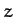-directed magnetic field of magnitude. The electron is situated at the origin atwith an initial-directed velocity of magnitude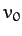. Show that the electron traces out a cycloid of the general form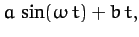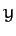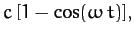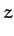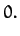Find the values of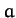,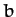,, and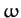, and sketch the electron's trajectory in the-plane when,, and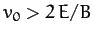.

2. A particle of massand chargemoves in the-plane under the influence of a constant amplitude rotating electric field which is such that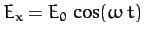and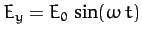. The particle starts at rest from the origin. Determine its subsequent motion. What shape is the particle's trajectory?

3. A particle of massslides on a frictionless surface whose height is a function ofonly: i.e.,. The functionis specified by the parametric equations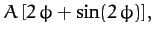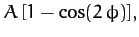where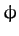is the parameter. Show that the total energy of the particle can be written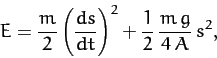where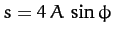. Deduce that the particle undergoes periodic motion whose frequency is amplitude independent (even when the amplitude is large). Demonstrate that the frequency of the motion is given by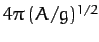.Next: Planetary Motion Up: Multi-Dimensional Motion Previous: Charged Particle Motion in
Richard Fitzpatrick 2011-03-31